@devilogic 2017-05-12T12:24:27.000000Z 字数 4551 阅读 1162

# 玩命的数据分析学习日志（三） - 神经网络探讨1

devilogic

## 神经网络ABC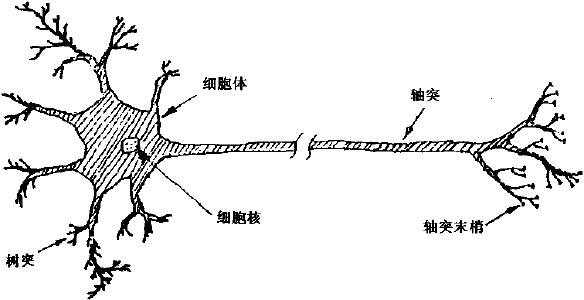### 单个神经元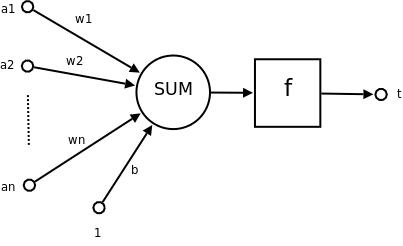### 前向反馈网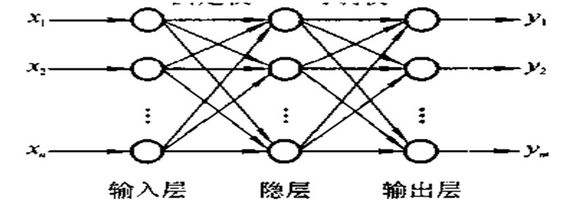### 三体1. 输入 -> 特征 -> 输入变量 -> 观察到的特征
2. 神经元 -> 权重 -> 方程的个数 -> 特征对输出的贡献
3. 输出 -> 解值 -> 解方程组方程的个数 -> 预测值
4. 隐藏层数 -> 尝试不同线性空间 -> 方程组的个数 -> 尝试找寻那个神秘的变量或者剔除提纯特征集

#### 让我们先来探讨一下决策树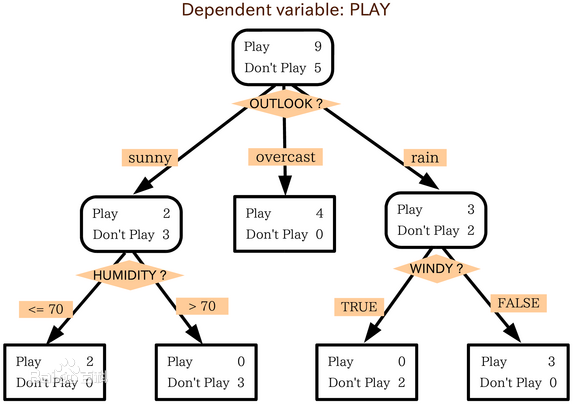### 一个找解的过程 - 梯度下降法(gradient descent)

1.导数为正则说明比正解大
2.导数为负则说明比正解小

• $x_{ji}$ 单元$j$的第$i$个输入
• $w_{ji}$ 与单元$j$的第$i$个输入相关联的权值
• $net_j = \sum_iw_{ji}x_{ji}$ 单元$j$的输入的加权和
• $o_j$ 单元$j$计算出的输出
• $t_j$ 单元$j$的目标输出
• $\sigma$ sigmoid函数
• outputs 网络最后一层的单元的集合
• Downstream{j} 单元的直接输入中包含单元$j$的输出的单元的集合

#### 误差

$E_d(\overrightarrow{w}) \equiv \frac{1}{2}\sum_{k \in outputs}(t_k - o_k)^2$

## 泛化上述公式

$o_j(1-o_j)$sigmod的导数，那么如果我们将这个替换成任意激励函数的导函数则有:

## 总结

1. 计算输出层的误差并生成梯度
2. 使用梯度对每个隐藏层的每个神经元进行求导并调节

## 当$k$无限大时

$k$无限大，就是说训练已经收敛时，那么最良好的情况是$w_j(k+1) = w_j(k)$，则说明了梯度为$0$，那么：

## 推荐书籍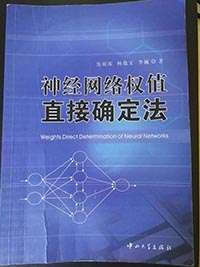## 感谢与抱歉• 私有
• 公开
• 删除